# Convolution of functions

(diff) ← Older revision | Latest revision (diff) | Newer revision → (diff)

$f$ and $g$ belonging to $L(-\infty, +\infty)$

The function $h$ defined by \begin{equation} h(x) = \int\limits_{-\infty}^{+\infty}f(x-y)g(y)\,dy = \int\limits_{-\infty}^{+\infty}f(y)g(x-y)\,dy; \end{equation} it is denoted by the symbol $f*g$. The function $f*g$ is defined almost everywhere and also belongs to $L(-\infty, +\infty)$.

## Contents

#### Properties

The convolution has the basic properties of multiplication, namely, \begin{equation} f*g = g*f, \end{equation} \begin{equation} (\alpha_1f_1 + \alpha_2f_2)*g = \alpha_1(f_1*g) + \alpha_2(f_2*g), \quad \alpha_1, \alpha_2 \in \mathbb{R}, \end{equation} \begin{equation} (f*g)*h = f*(g*h) \end{equation}

for any three functions in $L(-\infty, \infty)$. Therefore, $L(-\infty, \infty)$ with the usual operations of addition and of multiplication by a scalar, with the operation of convolution as the multiplication of elements, and with the norm \begin{equation} \|f\| = \int\limits_{-\infty}^{\infty}|f(x)|\, dx, \end{equation} is a Banach algebra (for this norm $\|f*g\|\leq \|f\|\cdot \|g\|$).

If $F[f]$ denotes the Fourier transform of $f$, thenand this is used in solving a number of applied problems.

Thus, if a problem has been reduced to an integral equation of the form(*)

where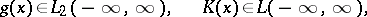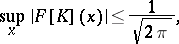then, under the assumption that, by applying the Fourier transformation to (*) one obtains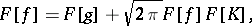hence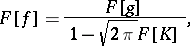and the inverse Fourier transformation yields the solution to (*) as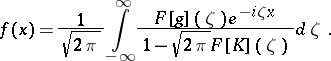The properties of a convolution of functions have important applications in probability theory. Ifandare the probability densities of independent random variablesand, respectively, thenis the probability density of the random variable.

The convolution operation can be extended to generalized functions (cf. Generalized function). Ifandare generalized functions such that at least one of them has compact support, and ifis a test function, thenis defined by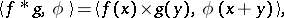where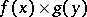is the direct product ofand, that is, the functional on the space of test functions of two independent variables given byfor every infinitely-differentiable function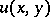of compact support.

The convolution of generalized functions also has the commutativity property and is linear in each argument; it is associative if at least two of the three generalized functions have compact supports. The following equalities hold: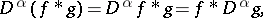whereis the differentiation operator andis any multi-index,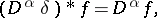in particular,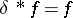, wheredenotes the delta-function. Also, if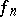,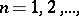are generalized functions such that, and if there is a compact setsuch that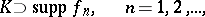then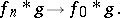Finally, ifis a generalized function of compact support andis a generalized function of slow growth, then the Fourier transformation can be applied to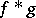, and again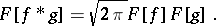The convolution of generalized functions is widely used in solving boundary value problems for partial differential equations. Thus, the Poisson integral, written in the form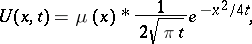is a solution to the thermal-conductance equation for an infinite bar, where the initial temperaturecan be not only an ordinary function but also a generalized one.

Both for ordinary and generalized functions the concept of a convolution carries over in a natural way to functions of several variables; then in the aboveandmust be regarded as vectors fromand not as real numbers.